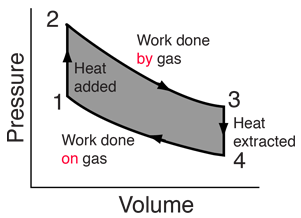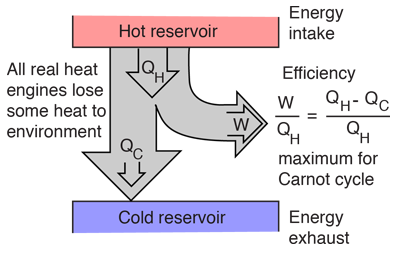# Heat Engine Cycle

A heat engine typically uses energy provided in the form of heat to do work and then exhausts the heat which cannot be used to do work. Thermodynamics is the study of the relationships between heat and work. The first law and second law of thermodynamics constrain the operation of a heat engine. The first law is the application of conservation of energy to the system, and the second sets limits on the possible efficiency of the machine and determines the direction of energy flow.

 Analysis of a simple cycle.

Heat engines are typically illustrated on a PV diagramHeat engines such as automobile engines operate in a cyclic manner, adding energy in the form of heat in one part of the cycle and using that energy to do useful work in another part of the cycle.
Index

Heat engine concepts

 HyperPhysics***** Thermodynamics R Nave
Go Back

# PV Diagrams

Pressure-Volume (PV) diagrams are a primary visualization tool for the study of heat engines. Since the engines usually involve a gas as a working substance, the ideal gas law relates the PV diagram to the temperature so that the three essential state variables for the gas can be tracked through the engine cycle. Since work is done only when the volume of the gas changes, the diagram gives a visual interpretation of work done. Since the internal energy of an ideal gas depends upon its temperature, the PV diagram along with the temperatures calculated from the ideal gas law determine the changes in the internal energy of the gas so that the amount of heat added can be evaluated from the first law of thermodynamics. In summary, the PV diagram provides the framework for the analysis of any heat engine which uses a gas as a working substance.For a cyclic heat engine process, the PV diagram will be a closed loop. The area inside the loop is a representation of the amount of work done during a cycle. Some idea of the relative efficiency of an engine cycle can be obtained by comparing its PV diagram with that of a Carnot cycle, the most efficient kind of heat engine cycle.
Index

PV diagram concepts

Heat engine concepts

 HyperPhysics***** Thermodynamics R Nave
Go Back

# Heat Engines

A heat engine typically uses energy provided in the form of heat to do work and then exhausts the heat which cannot be used to do work. Thermodynamics is the study of the relationships between heat and work. The first law and second law of thermodynamics constrain the operation of a heat engine. The first law is the application of conservation of energy to the system, and the second sets limits on the possible efficiency of the machine and determines the direction of energy flow.General heat engines can be described by the reservoir model (left) or by a PV diagram (right)

Index

PV diagram concepts

Heat engine concepts

 HyperPhysics***** Thermodynamics R Nave
Go Back

# Energy Reservoir Model

One of the general ways to illustrate a heat engine is the energy reservoir model. The engine takes energy from a hot reservoir and uses part of it to do work, but is constrained by the second law of thermodynamics to exhaust part of the energy to a cold reservoir. In the case of the automobile engine, the hot reservoir is the burning fuel and the cold reservoir is the environment to which the combustion products are exhausted.The efficiency expression given is a general one, but the maximum efficiency is limited to that of the Carnot cycle. This limitation is often called the thermal bottleneck.

Another common way to characterize heat engines is by a PV diagram

Index

Heat engine concepts

 HyperPhysics***** Thermodynamics R Nave
Go Back